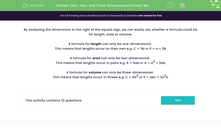# One-, Two- and Three-Dimensional Formula: Which is It?

In this worksheet, students decide whether a given formula is one-dimensional, two-dimensional or three-dimensional.Key stage:  KS 3

Curriculum topic:   Algebra

Curriculum subtopic:   Use Standard Mathematical Formulae

Difficulty level:#### Worksheet Overview

By analysing the dimensions to the right of the equals sign, we can easily say whether a formula could be for length, area or volume.

A formula for length can only be one-dimensional.

This means that lengths occur on their own e.g. C = 3d or P = a + 2b

A formula for area can only be two-dimensional.

This means that lengths occur in pairs e.g. A = 3ab or A = a2 + 2ab

A formula for volume can only be three-dimensional.

This means that lengths occur in threes e.g. C = 5d3 or P = abc + 2a2b

### What is EdPlace?

We're your National Curriculum aligned online education content provider helping each child succeed in English, maths and science from year 1 to GCSE. With an EdPlace account you’ll be able to track and measure progress, helping each child achieve their best. We build confidence and attainment by personalising each child’s learning at a level that suits them.

Get started